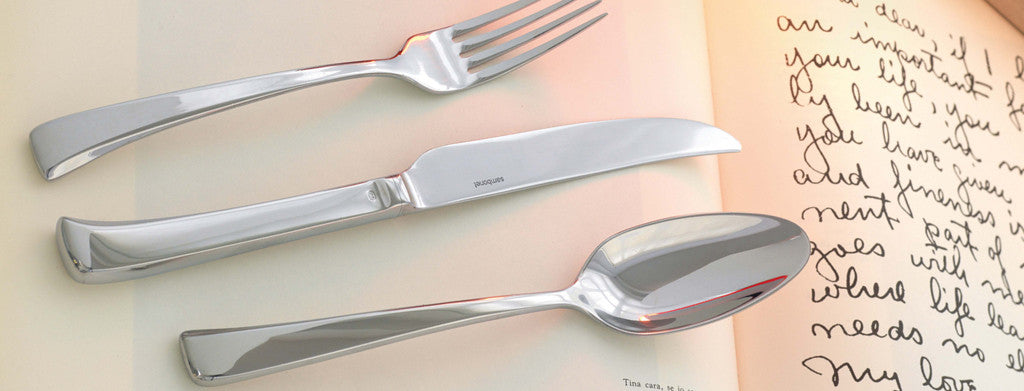Shopping Cart

### Refine

View all

#### Price# Cutlery£3.40 each (ex VAT)£2.52 each (ex VAT)£2.52 each (ex VAT)£3.95 each (ex VAT)£1.43 each (ex VAT)£3.89 each (ex VAT)£4.30 each (ex VAT)£6.15 each (ex VAT)£6.76 each (ex VAT)£12.24£5.48 each (ex VAT)£3.46 each (ex VAT)£1.71 each (ex VAT)£3.98 each (ex VAT)£3.40 each (ex VAT)£7.39 each (ex VAT)£0.31 each (ex VAT)£0.55 each (ex VAT)£3.95 each (ex VAT)£4.30 each (ex VAT)£2.52 each (ex VAT)£3.23 each (ex VAT)£9.48 each (ex VAT)£0.34 each (ex VAT)£0.31 each (ex VAT)£0.53 each (ex VAT)£0.52 each (ex VAT)£0.76 each (ex VAT)£0.59 each (ex VAT)£0.85 each (ex VAT)£4.83 each (ex VAT)£2.87 each (ex VAT)£2.46 each (ex VAT)£3.89 each (ex VAT)£0.55 each (ex VAT)£0.53 each (ex VAT)£0.53 each (ex VAT)£0.34 each (ex VAT)£0.55 each (ex VAT)£0.87 each (ex VAT)£1.43 each (ex VAT)£1.21 each (ex VAT)£1.21 each (ex VAT)£0.73 each (ex VAT)£1.03 each (ex VAT)£1.43 each (ex VAT)£0.86 each (ex VAT)£0.76 each (ex VAT)